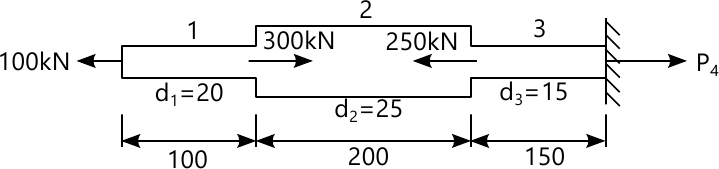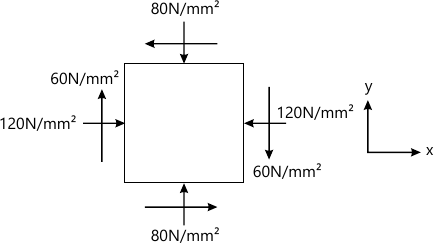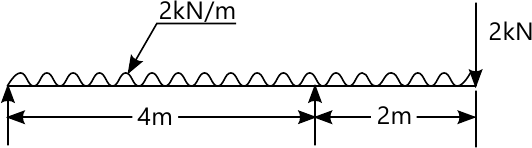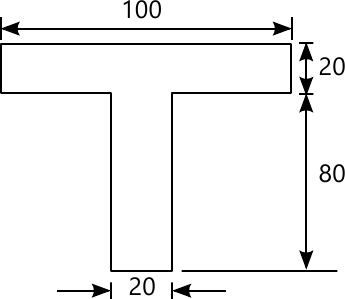MORE IN Mechanics of Materials
VTU Mechanical Engineering (Semester 3)
Mechanics of Materials
January 2013
Total marks: --
Total time: --
INSTRUCTIONS
(1) Assume appropriate data and state your reasons
(2) Marks are given to the right of every question
(3) Draw neat diagrams wherever necessary

1 (a) Define:
Duclicity
true stress
principle of super position
6 M
1 (b) Determine the stresses In various segment of the circular bar shown in fig.Q1 b

Compter its total elogation assuming Young modulus of steel to be 195GPa.
Dtermine the lenghth of the middle segment so the the bar length does not change under the applied8 M
1 (c) Derive an expression for the extension of uniformly tapering rectangular bar subjected to axial load P
6 M

2 (a) A.C.I.flat ,300mm long ,50mm wide and 30 mm thick is acted upon by the following forces .25kN tensile in the direction of length ,350 kN compressive in the direction of width and 200KN tensile in the dirction of thickness.Detemine
change in volume of the flat
modulus of rigidity
Bulk modulus
Take E=140GN/m2 and \dfrac{1}{m}=0.25
10 M
2 (b) A steel bar is placed between two copper, each having the same area and length L as the steel bar at 15°C, at this stage ,they are rigidly connected together at both the ends. the length of composite bar is also L.when the tempreture is raised to 3157deg;C the length of the Take Es=2.1×105N/mm2
Ec=1×105N/mm2
αs=0.000012 per°C
αs=0.0000175 per°C
10 M

3 (a) The state of stress in a two dimenssional stressed body is shown in Fig .Q.3.Determine the principle planes principle stresses .maximum shear stress and their planes .Also draw the Mmohr's to verify the result obatined analytically .Indiactes all the above plane by a skecth20 M

4 (a) A simply supposed beam of spam L .carries a point load W at mid-span .Find the strain energy stored by beam
5 M
4 (b) Derive an expression for circumferential stress for thin cylinder
5 M
4 (c) A Cylindrical pressure vessel has and outer redil of 200mm and 250mm respectively The material of the cylinder has an allowable normal stress of 75 MN/mm2,Determine the maximum internal pressure that can be applied and draw a sketch of radial pressure circumference stress disttribution
10 M

5 (a) A cantilever of length 2.0 m carries a uniformly distributed load of 1kN/m run over length of 1.5 m from the free end.Draw the shear force and bending moment diagram for the cantilever
6 M
5 (b) Draw shear force and bending moment diagrams for the overhnaiging beam carrying uniformaly distributed load of 2kN/m over the entire length and a point shown ,as shown in fig.Q.5(b)Loacte of point of contra.14 M

6 (a) A cast is beam a T-section as shown in fig.Q6(a).The beam is simply supported on a span of 8m .The carries a uniformaly distributed load of 1.5 kn/m length on the entire span.Detrmine the maximum tensile and maxmium compressive stresses10 M
6 (b) A beam of reactangular section of width 'b' and depth is subjected to shear force.Draw the fig showing the shear stress variation across the section .Also show that maximum shear is 1.5 times the average shear stress
10 M

7 (a) A canilever of length 2.5m carrie sa uniformaly distributed load of 16.4kN/m over the entire length .if the of inertia of the beam is 7.95×107mm4 and the value of E=2 ×105,determine the deflaction at the free end .Derive the equation used
10 M
7 (b) A beam of length 6m is simply supported at its ends and carries two points loads of 48kN a d 40kN at a distance 0f 1 m and 3m respectively from the left support Find: